s-tefan

•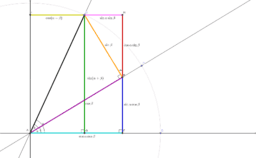The addition formula for sine and cosine

Activity

s-tefan

•Demonstration of the law of cosines

Activity

s-tefan

•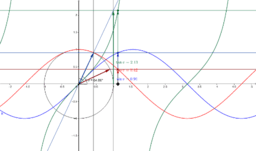The unit circle and graphs of trigonometric functions

Activity

s-tefan

•The unit circle and graphs of trigonometric functions

Activity

s-tefan

•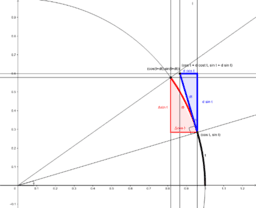Derivatives of sine and cosine

Activity

s-tefan

•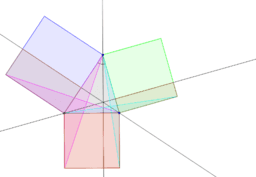The Cosine Theorem

Activity

s-tefan

•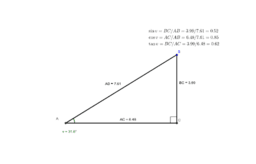Trigonometry in a right-angled triangle

Activity

s-tefan

•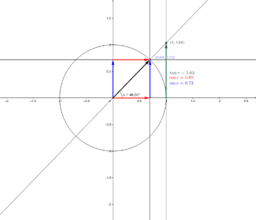Trigonometry in a unit circle

Activity

s-tefan

•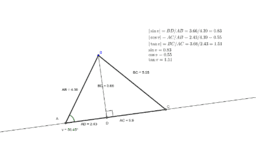Trigonometry in a general triangle

Activity

s-tefan

•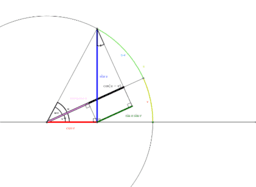Activity

s-tefan